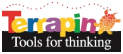Terrapin Resources

# Snowflakes

by Bill SpezeskiThis project uses a fractal formula from “Logo Models and Methods for Problem Solving” to create six-sided snowflakes by altering one variable in the formula.

This project illustrates

• illustrating fractals in Logo
• changing variables in a formula to change designs
• using FOR command to repeat with a counter

#### Snowflakes.lgo

``````; from Chapter 8 of Models and Methods for Problem Solving
; by Bill Spezeski

TO SETUP.FRAC :NPTS :M :R :SCALE :TILT
CS FS WINDOW
MAKE "ANGLE :M*(360/:NPTS)
MAKE "LSIDE :R*(SIN :ANGLE/2)
SETH 0
PU FD :R/2 PD
RT 90 + :ANGLE/2 + :ADJUST
DRAW.FRAC :NPTS :LSIDE
END

TO DRAW.FRAC :NPTS :LSIDE
IF :LSIDE < 10 STOP
REPEAT :NPTS [FD :LSIDE DRAW.FRAC :NPTS :LSIDE * :SCALE RT :ANGLE]
END

TO SETUP.FRACT :NPTS :M :R :SCALE :TILT :LEVEL
CS SETBG "NAVY SETPC "WHITE FS WINDOW
MAKE "ANGLE :M * (360/:NPTS)
MAKE "LSIDE :R * (SIN :ANGLE /2)
MAKE "ADJUST (180 - :ANGLE) * :TILT + (360 - :ANGLE) *(1- :TILT)
SETH 0
PU FD :R /2 PD
DRAW.FRACT :NPTS :LSIDE :LEVEL
END

TO DRAW.FRACT :NPTS :LSIDE :LEVEL
IF :LEVEL = 0 [STOP]